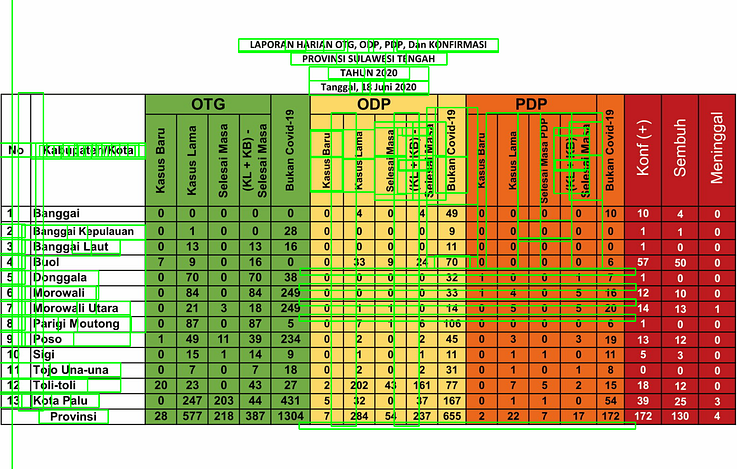## Magnetometer Calibration Using Levenberg-Marquardt Algorithm

Recently I worked on a magnetometer calibration method. This method is based on Levenberg-Marquardt Algorithm (LMA), a non-linear least-squares optimization algorithm. The method is implemented on ArduPilot and PX4, an open-source flight controller firmware.

I have to admit, formulizing mathematical notion from code is not straightforward. I spent several days to learn from LMA basic and finally understanding the sphere fit and ellipsoid fit algorithm.

In case you are wondering about the mathematical part, I write the formulation of the algorithm on PDF since it can’t be viewed on WordPress (unless I pay more for the plugin).

## Python vs Julia: Speed Test on Fibonacci Sequence

Recently MIT released a course on Computational Thinking with code 18.S191 and it is available on YouTube. I can code in C++ and Python, so the founder’s claim that this code is as fast as C and as easy as Python gains my interest.

## Introduction to Julia

Julia is created in 2009 and first introduced to public in 2012. The developers aimed for scientific computing, machine learning, data mining, and large-scale linear algebra. We might have heard this application on Python, but Julia gives advantages to programmer compared to Python.

Continue reading Python vs Julia: Speed Test on Fibonacci Sequence

## Text Extraction from a Table Image, using PyTesseract and OpenCV

Extracting text from an image can be exhausting, especially when you have a lot to extract. One commonly known text extraction library is PyTesseract, an optical character recognition (OCR). This library will provide you text given an image.

PyTesseract is really helpful, the first time I knew PyTesseract, I directly used it to detect some a short text and the result is satisfying. Then, I used it to detect text from a table but the algorithm failed perform.Figure 1. Direct use of PyTesseract to Detect Text in a Table

Figure 1 depicts the text detection result, with green boxes enclosing the detected words. You may realized that most of the text can’t be detected by the algorithm, especially numbers. In my case, these numbers are the essentials of the data, giving me value of daily COVID-19 cases from a local government in my hometown. So, how extract these information?

## Getting Started

When writing an algorithm, I always try to think as if I’m teaching the algorithm the way humans do. This way, I can easily put the idea into more detailed algorithms.

When you’re reading a table, the first thing you might notice is the cells. A cell might be separated from another cell using a border (lines), which can be vertical or horizontal. After you identify the cell, you proceed to read the information within. Converting it into algorithm, you may divide the process into three processes, namely cells detection, region of interest (ROI) selection, and text extraction.

Continue reading Text Extraction from a Table Image, using PyTesseract and OpenCV